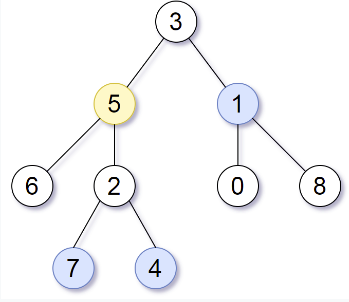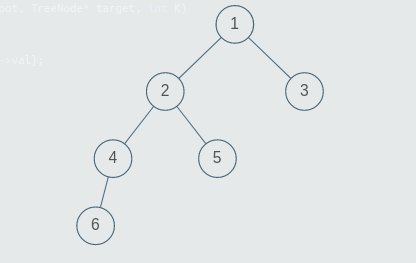New update is available. Click here to update.

# Print Nodes at Distance K From a Given Node

Contributed by
Prashansa
Last Updated: 23 Feb, 2023
Hard0/120
Avg time to solve 20 mins
Success Rate 80 %Share13 upvotes

## Problem Statement

#### Note:

``````1. A binary tree is a tree in which each node has at most two children.
2. The given tree will be non-empty.
3. The given tree can have multiple nodes with the same value.
4. If there are no nodes in the tree which are at distance = K from the given node, return an empty list.
5. You can return the list of values of valid nodes in any order. For example if the valid nodes have values 1,2,3, then you can return {1,2,3} or {3,1,2} etc.
``````
Detailed explanation ( Input/output format, Notes, Images )##### Constraints:
``````1 <= T <= 100
1 <= N <= 3000
0 <= K <= 3000
0 <= nodeValue <= 3000

Where nodeValue donates the value of the node.

Time Limit: 1 sec
``````
##### Sample Input 1 :
``````1
3 5 1 6 2 0 8 -1 -1 7 4 -1 -1 -1 -1 -1 -1 -1 -1
5
2
``````
##### Sample Output 1 :
``````7 4 1
``````
##### Explanation For The Sample Output 1:``````Target Node is 5. Nodes at distance 1 from 5 are {6, 2, 3} and nodes at distance 2 are {7, 4, 1}.
``````
##### Sample Input 2:
``````1
1 2 3 4 5 -1 -1 6 -1 -1 -1 -1 -1
5
3
``````
##### Sample Output 2:
``````6 3
``````
##### Explanation For The Sample Output 2:``````The tree will be generated as above. The target node is 5 and K = 3. The nodes at distance 1 from node 5 are {2}, nodes at distance 2 from node 5 are {1, 4} and nodes at distance 3 from node 5 are {6, 3}.
``````AutoConsole